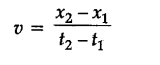# IN the given position-time graph of a non-uniform motion what will be the average velocity between \${{x}_{1}}\$ and \${{x}_{2}}\$?

In the given position-time graph of a non-uniform motion what will be the average velocity between {{x}_{1}} and {{x}_{2}} ?Average velocity is the slope of the line {{x}_{1}}, {{x}_{2}} , i.e.,